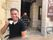cancel
Showing results for
Did you mean:# Introduction

Cbind is a function in R that allows you to bind columns / vectors or tables, not by joining them using a column, but by adding the column to the table in the existing order.

# Solution

There are use cases where we would like to use this function in Power Query as well. For example when preparing data for a custom Visual in Power BI, since they only accept one dataset.

The Power M code looks like this:

``````(listoftables, prefixvar) =>
let

//first column (original list, holds the XY positions, so we rename this column
//RenameCol = List.Transform(AddIndex, each Table.RenameColumns(_,{{"Column1", "xy"}})),
//each table with points had a empty record
//RemoveExptyRows = List.Transform(RenameCol, each Table.SelectRows(_, each [xy] <> "") ),
// Name the dataset
prefix = Table.TransformColumns(Changed_Type,{{"ColName", each prefixvar & _ & "_"}}),

// get the max index per pointset
max = Table.AddColumn(prefix, "m",  each List.Max(_[Column1][Index])),

//generate a list that will represent our main column to which we will cbind the others
maxofmax = List.Max(max[m]),
l = List.Generate(()=>0, each _ < maxofmax, each _ + 1 ),
Index_ConvertToTable = Table.FromColumns({l},
type table[ Index = Int64.Type ]),

//Convert to Table with our pointsets to a list, also contains the name of the set! (second column)
TableRows = Table.ToRows(max)
, // we will iterate TableRows, to current will reflect to row being interated,
//Index_ConvertToTable reflects the first state
JoinTables = List.Accumulate(TableRows,Index_ConvertToTable,(state,current) =>
let
Joined = Table.NestedJoin(state,{"Index"},current{0},{"Index"},"JoinedTableColumn",JoinKind.LeftOuter),
ColumnNames1 = List.Difference(Table.ColumnNames(current{0}),{"Index"}), //All Column names other than index
ColumnNames2 = List.Transform( ColumnNames1, each (current{1}) & _) , //All these columns with a prefix, being the name of the dataset
Expanded = Table.ExpandTableColumn(Joined,"JoinedTableColumn",ColumnNames1, ColumnNames2) //These columns exanded and renames to the prefix name
in
Expanded //The joined dataset becomes the new state.
),
ChangeType = Table.TransformColumnTypes(JoinTables,{{"Index", Int64.Type}}),
Sort = Table.Sort(ChangeType,{{"Index", Order.Ascending}})
in Sort``````

This function accepts two parameters, the first one being a list of tables, and the second one a prefix you want to give the table-name (which will be numbered).

In M you can create a list of tables like this:

`{ table1, table2 }`

You can run a test by connecting Visual Studio (with the Power Query SDK installed) to this github repository. When you run the code you will see these results:

In this code, you will notice some code that decompresses a string to a table. In this article, you can find more info on how to use this compression function.

## Code walkthrough

The code consists of 3 main parts,

• Adding an index column to each table.
• Getting the number of rows per table. Using the maximum number of rows, create a table that is that long (with an index column.
• Using List.Accumulate we recursively join each table to the created index table.

Hope this is of value to you!